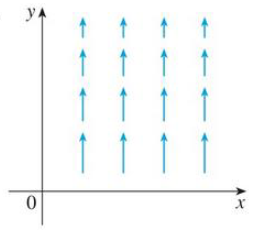Chapter 16.5, Problem 9E

Chapter
Section
Textbook Problem

The vector field F is shown in the xy-plane and looks the same in all other horizontal planes. (In other words, F is independent of z and its z-component is 0.)(a) Is div F positive, negative, or zero? Explain.(b) Determine whether curl F = 0. If not, in which direction does curl F point?9.(a)

To determine

To find and explain: The div F.

Explanation

Formula used:

Consider the standard equation of a divergence of vector field for F=Pi+Qj+Rk

divF=Px+Qy+Rz (1)

As F is independent of z-component (R=0) is Rx=Ry=Rz=0 .

From Figure, the x-component of each vector is 0 (P=0) , hence Px=Py=Pz=0

and Q dec

(b)

To determine

Whether curl F=0 or not.

Still sussing out bartleby?

Check out a sample textbook solution.

See a sample solution

The Solution to Your Study Problems

Bartleby provides explanations to thousands of textbook problems written by our experts, many with advanced degrees!

Get Started

For what value of k does the equation e2x=kx have exactly one solution?

Single Variable Calculus: Early Transcendentals, Volume I

For the following set of scores, find the value of each expression: a. X b. (X)2 c. X2 d. (X +3)

Essentials of Statistics for The Behavioral Sciences (MindTap Course List)

Finding a Limit In Exercises 1128, find the limit. limx2t+2t24

Calculus: Early Transcendental Functions (MindTap Course List)

The gradient vector field for is:

Study Guide for Stewart's Multivariable Calculus, 8th

356dx= a) 2 b) 8 c) 6 d) 12

Study Guide for Stewart's Single Variable Calculus: Early Transcendentals, 8th

Construct the tangent line to P at point X.

Elementary Geometry for College Students